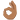# Fourier's Trick and calculation of Cn

• I
I understand that the solutions to the time-independent Schrodinger equation are complete, so a linear combination of the wavefunctions can describe any function (i.e. ##f(x) = \sum_{n = 1}^{\infty}c_n\psi_n(x) = \sqrt{\frac{2}{a}} \sum_{n=1}^{\infty} c_n\sin\left(\frac{n\pi}{a}x\right)## for the infinite well case).

I don't understand how using Fourier's trick allows you to calculate ##c_n##. Also, I don't understand how ##\sum_{n=1}^{\infty}c_n\delta_{mn} = c_m##. I get that the Kronecker delta comes out of the fact that ##\psi_m^\ast(x)## and ##\psi_n(x)## are orthogonal. I understand that when ##m = n##, then the Kronecker delta equals 1, but how does this allow us to find ##c_m## and ##c_n## .
$$\int_{0}^{a} \psi_m^\ast(x) f(x)\mathrm dx = \sum_{n=1}^{\infty}c_n \int_{0}^{a} \psi_m^\ast (x)\psi_n(x)\mathrm dx = \sum_{n=1}^{\infty}c_n\delta_{mn} = c_m$$

Related Quantum Physics News on Phys.org
BvU
Homework Helper
2019 Award
I don't understand how using Fourier's trick allows you to calculate ##c_n##.

Also, I don't understand how ##\sum_{n=1}^{\infty}c_n\delta_{mn} = c_m##
That's because ##\delta=0## unless m = n; then it's 1.Fourier analysis is a bit more than just a 'trick'Fourier analysis is a bit more than just a 'trick'
I referred to it as a trick because that's what the material I was reading referred to it as. I'll take a look into Fourier transforms then.

Nugatory
Mentor
I referred to it as a trick because that's what the material I was reading referred to it as. I'll take a look into Fourier transforms then.
What was that material? You'll generally get better answers if you identify your sources - chances are that someone here is already familiar with it.

(I remember that exact phrase "Fourier's trick" appearing in a thread here a while back)

•BvU
It was from Griffiths, Introduction to quantum mechanics second edition chapter 2

BvU
Homework Helper
2019 Award
Found it on p 28. (and you are not responsible for his wording)
Now I'm puzzled about your question: you reproduce the line that works out ##c_m##, so that should answer it !?

(##c_n## does not come out, though! n is just a summation variable)

I understand the properties of the Kronecker delta, what I didn't understand was why ##c_m## came out. So, let me get this right. When applying the Kronecker delta to ##\sum_{n = 1}^{\infty} c_n##, you will get a coefficient value that both ##\psi_n## and ##\psi_m## share (due to the properties of ##\delta_{mn}##)?

What I misunderstood previously, was that I thought ##\psi_m## consisted of multiple coefficients, and so ##\delta_{mn}## when applied would work out the mutual coefficients between ##\psi_n## and ##\psi_m##, however only one coefficient (##c_m##) was stated.

phyzguy
Think of it like a dot product. If I have a 3D vector $\overrightarrow{V} = V_x \overrightarrow{e_x} + V_y \overrightarrow{e_y} + V_z \overrightarrow{e_z}$, and I take the dot product with a unit vector, I get $\overrightarrow{V} \centerdot \overrightarrow{e_x} = V_x$. this is because the unit vectors are orthogonal, so the dot product "picks off" the component along the x-axis. It's exactly the same in Fourier analysis, even though there are an infinite number of "unit vectors".
•Ah, that's a nice way to think about it